# Data Clustering Using Apache Spark

### This article looks at the analysis of cancer survival using K-means and Gaussian Mixture algorithms.

· Big Data Zone · Tutorial
Save
35.31K Views

## Introduction

Apache Spark is a cluster computing system with many application areas including structured data processing, machine learning, and graph processing. The online literature on Apache Spark framework is extensive. For example, see the official Apache Spark documentation, Introduction to Apache Spark, Big Data Processing in Spark, Apache Spark and artificial neural networks.

The focus of this article is MLlib, the Spark machine learning library. We will demonstrate three separate clustering algorithms in MLlib, K-Means, Bisecting K-Means and Gaussian Mixture, in an example for exploratory analysis of colorectal cancer survival.

The article is organized as follows. In the next section, we give an overview of cluster analysis. The following section discusses the example where we apply cluster analysis to colorectal cancer research data. In that example, we will cluster patients based on similarities between two features, ‘regional nodes positive’ and ‘stage group’, to analyze if the resulting clusters will imply duration of patient survival. We will show that average survival duration for patients in a cluster decreases as the cluster center has increasing ‘regional nodes positive’ and ‘stage group’. Then, we give concluding remarks. Finally, we provide a code review for each of the algorithms considered in the article.

## Cluster Analysis

Clustering is the task of assigning entities into groups based on similarities among those entities. The goal is to construct clusters in such a way that entities in one cluster are more closely related, i.e. similar to each other than entities in other clusters. As opposed to classification problems where the goal is to learn based on examples, clustering involves learning based on observation. For this reason, it is a form of unsupervised learning task.

There are many different clustering algorithms and a central notion in all of those is the definition of ’similarity’ between the entities that are being grouped. Different clustering algorithms may have different ways of measuring the similarity. In many clustering algorithms, another common notion is the so-called cluster center, which is a basis to represent the cluster. For example, in K-means clustering algorithm, the cluster center is the arithmetic mean position of all the points in that cluster.

As an exploratory data analysis tool, clustering has many application areas across various disciplines including social sciences, biology, medicine, business & marketing and computer sciences. Below are some examples.

• Use clustering to group entities based on certain features and then analyze if other features in each group are also close to each other. An example is grouping of tumor DNA microarray data based on gene expressions and then inferring if those groups will imply presence of certain types of cancer. Another example is grouping of patient data based on symptoms and signs and then deducing if those groups will differ from each other with respect to their therapeutic responsiveness or their prognosis.

The sample application considered in the article belongs to this category. We will cluster colorectal cancer patients based on ‘regional nodes positive’ and ‘stage group’ and then show that average survival duration for patients in a cluster decreases as the cluster center has increasing ‘regional nodes positive’ and ‘stage group’.
• Use clustering to group entities into a single cluster only to calculate a center. The center can later be utilized as a representative of all the entities in the group. An example is image compression where an image is first partitioned into small blocks of predetermined size and a cluster center is calculated for the pixels in each block. Then, the image is compressed where each block is replaced by an equally sized block approximated by block's center.
• Use clustering to reduce the amount of data for simplification of analysis. For example, grouping of patient laboratory results based on measured variables (qualitative or quantitative analytes or mathematically derived quantities) may help in understanding how lab data is structured for patients with certain diseases. Another example is segmentation i.e. pixel classification of medical images in order to aid in medical diagnosis.
• Use clustering to solve a classification problem. For example, MRI images of liver belonging to Cirrhosis patients at different stages and non-patients (i.e. free of Cirrhosis) are clustered into two groups, one representing cirrhotic and the other one representing non-cirrhotic cases. Then, MRI image of a new patient is compared to the cluster centers and based on proximity a prediction is made whether the patient is cirrhotic or not.

Below we give a high-level review of the three clustering algorithms considered in this article.

### K-means

K-means is among the most popular clustering algorithms. Number of clusters, k, is defined in advance. The centers of clusters and the data points in each cluster are adjusted via an iterative algorithm to finally define k clusters. There is an underlying cost minimization objective where the cost function is so-called Within-Cluster Sum of Squares (WCSS). Spark MLlib library provides an implementation for K-means clustering.

### Bisecting K-means

The bisecting K-means is a divisive hierarchical clustering algorithm and is a variation of K-means. Similar to K-means, the number of clusters must be predefined. Spark MLlib also provides an implementation for bisecting K-means algorithm.

### Gaussian Mixture

The Gaussian mixture clustering algorithm is based on the so-called Gaussian Mixture Model for assembling the clusters. The Apache Spark implementation for that algorithm utilizes an expectation maximization algorithm. Similar to K-means and bisecting K-means, the Gaussian mixture clustering algorithm implementation by Spark requires a predefined number of clusters.

### Determining Number of Clusters

There are certain types of clustering techniques that do not require number of clusters in advance.  On the other hand, common to all three algorithms, K-means, bisecting K-means and Gaussian mixture discussed above, the number of clusters must be determined in advance and supplied to the algorithm as a parameter. Hence, determining the number of clusters is a separate problem to solve. In this article, we used a heuristic approach based on Elbow Method. Starting from k := 2 clusters, we ran the K-means algorithm for the same data set by increasing k and observing the value of cost function WCSS. At some point, a big drop in cost function was observed but then the improvement became marginal with increasing k. As suggested in this cluster analysis lecture notes, we picked the k after the last big drop of WCSS. (We will explain this with an example later.)

## Clustering of Colorectal Cancer Patient Data

We will apply each of the three clustering algorithms discussed above to SEER (National Cancer Institute Surveillance, Epidemiology, and End Results Program) colorectal cancer statistics. For this purpose, we use the colorectal cancer data file from SEER 1973-2012 (November 2014 Submission) database. The data file has a fixed width ASCII file format where each row corresponds to the record of a unique patient and individual data fields (features) in each record are identified by their column positions. (For further details of the file format see this reference.)

For each patient record, we are interested in 3 data fields, that are all categorical, i.e. taking discrete values belonging to a known set, and are all integer types.

 Feature Name Explanation 1 Regional Nodes Positive Number of regional lymph nodes found to contain metastases in pathology test. 2 Derived AJCC Stage Group Stage (level of progression) of cancer in tumor determined according to American Joint Committee on Cancer (AJCC) staging system 6-th edition.0 - 2 correspond to stage 010 - 22 correspond to stage 130 - 43 correspond to stage 250 - 63 correspond to stage 370 - 74 correspond to stage 4 3 Survival Months Patient’s survival in months after diagnosis.
##### Table 1. Features used in data analysis.

We pre-processed the original data file as follows:

• We removed records that supplied 'unknown', 'not examined' or white space as the corresponding value for the considered features.
• To avoid 'survival months' of a record being artificially short due to early termination of observation period, we retained only those records with an observation period of at least 4 years.
• We eliminated records of patients who expired due to reasons other than colorectal cancer.

### Approach

We will apply each algorithm to cluster patient data based on features 1 and 2, i.e. ‘regional nodes positive’ and ‘derived AJCC stage group’ (shortly, ‘stage group’). Then, we will examine if those clusters imply any results in terms of ‘survival months’. We will show that, in general, as ‘regional nodes positive’ and ‘stage group’ of a cluster center increase, the average ‘survival months’ of the patients in the cluster decreases.

In real applications, the number of features in cluster data could be quite large. The clustering example for analyzing tumor DNA microarray data based on gene expressions consists of ~ 7,000 features. The simple example considered in this article uses only two features. This makes it easy to visually interpret the results in 2-dimensional graphs.

Before presenting the results let us discuss how we determined number of clusters. As shown below, we calculated the cost function WCSS as a function of number of clusters for K-means algorithm applied to patient data based on features 1 and 2. Observe that a ‘big drop’ occurs when k = 4. As discussed previously, we chose number of clusters to be 5, which is the one after the last big drop.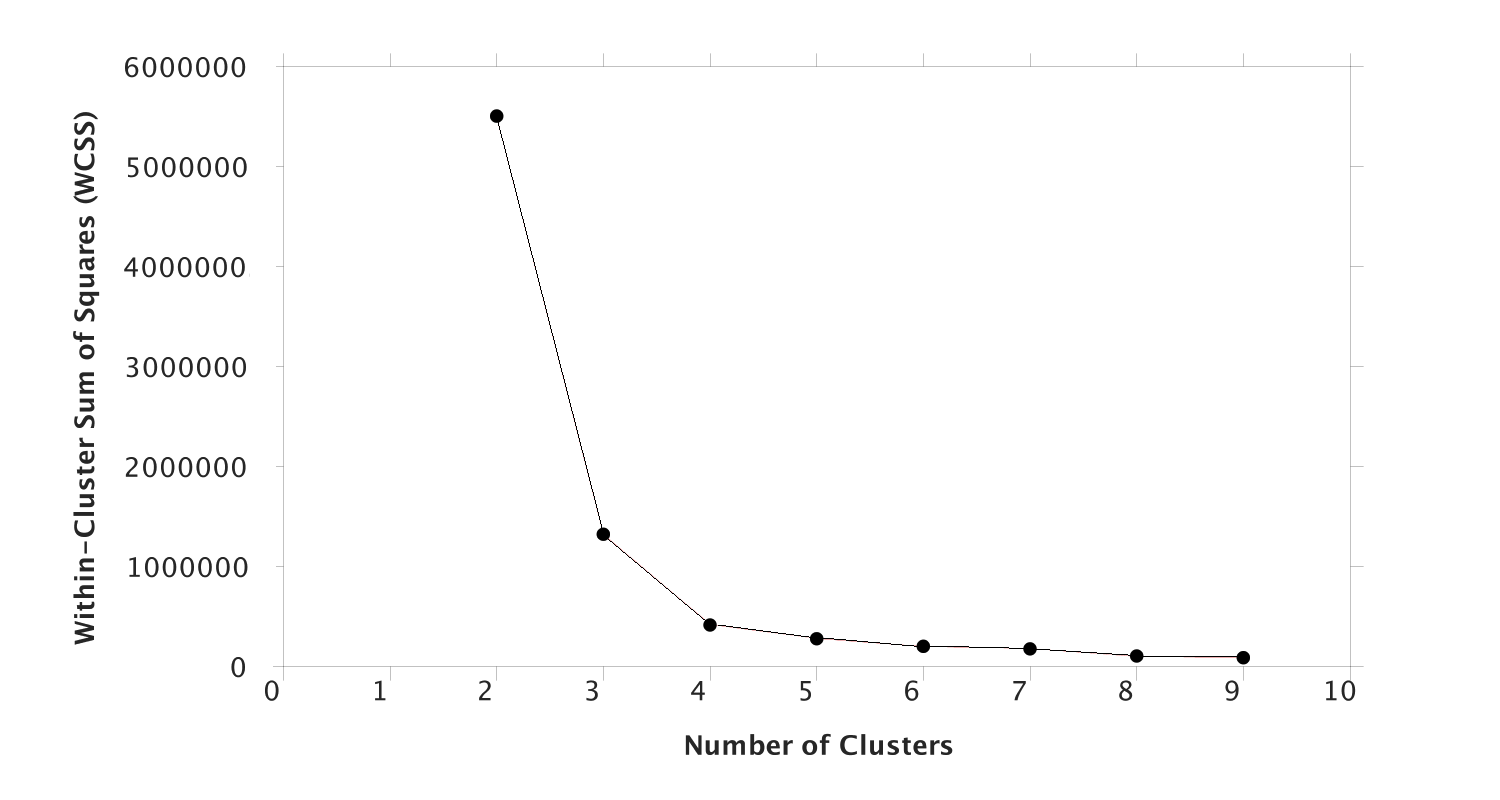### Results

Below, we show the clusters obtained by K-means, bisecting K-means and Gaussian mixture algorithms. Clusters are numbered 0 through 4. In each graph, cluster data points are shaped as circles. Each data point is represented by ‘stage group’ in horizontal axis and ‘regional nodes positive’ in vertical axis. Points in each cluster are colored differently. The cluster centers are black diamond shaped with a number indicating which cluster they belong to. Under each graph, there is a table describing the color of points in each cluster, number of patients in the cluster and average survival months of the patients in that cluster. For ease of interpretation, we numbered the clusters so that as the number increases, average survival months of the patients in the cluster decreases. In other words, average survival months for patients in cluster 0 is the highest whereas average survival months for patients in cluster 4 is the lowest.

 K-means Bisecting K-means Gaussian mixture Cluster Color #patients Average Survival Months #patients Average Survival Months #patients Average Survival Months 0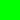6,404 72 6,404 72 16,070 68 1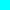9,660 66 9,660 66 6,553 61 29,731 58 8,009 60 3,481 49 3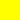4,345 27 2,036 44 4,559 27 4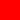648 25 4,679 26 125 16
##### Table 2. Description of clusters created via K-means, bisecting K-means and Gaussian mixture algorithms.

Note: Data in each graph corresponds to a total of 30,788 patients. Because multiple patients may have the same pair of ‘regional nodes positive’ and ‘stage group’, there are not as many data points.

### K-means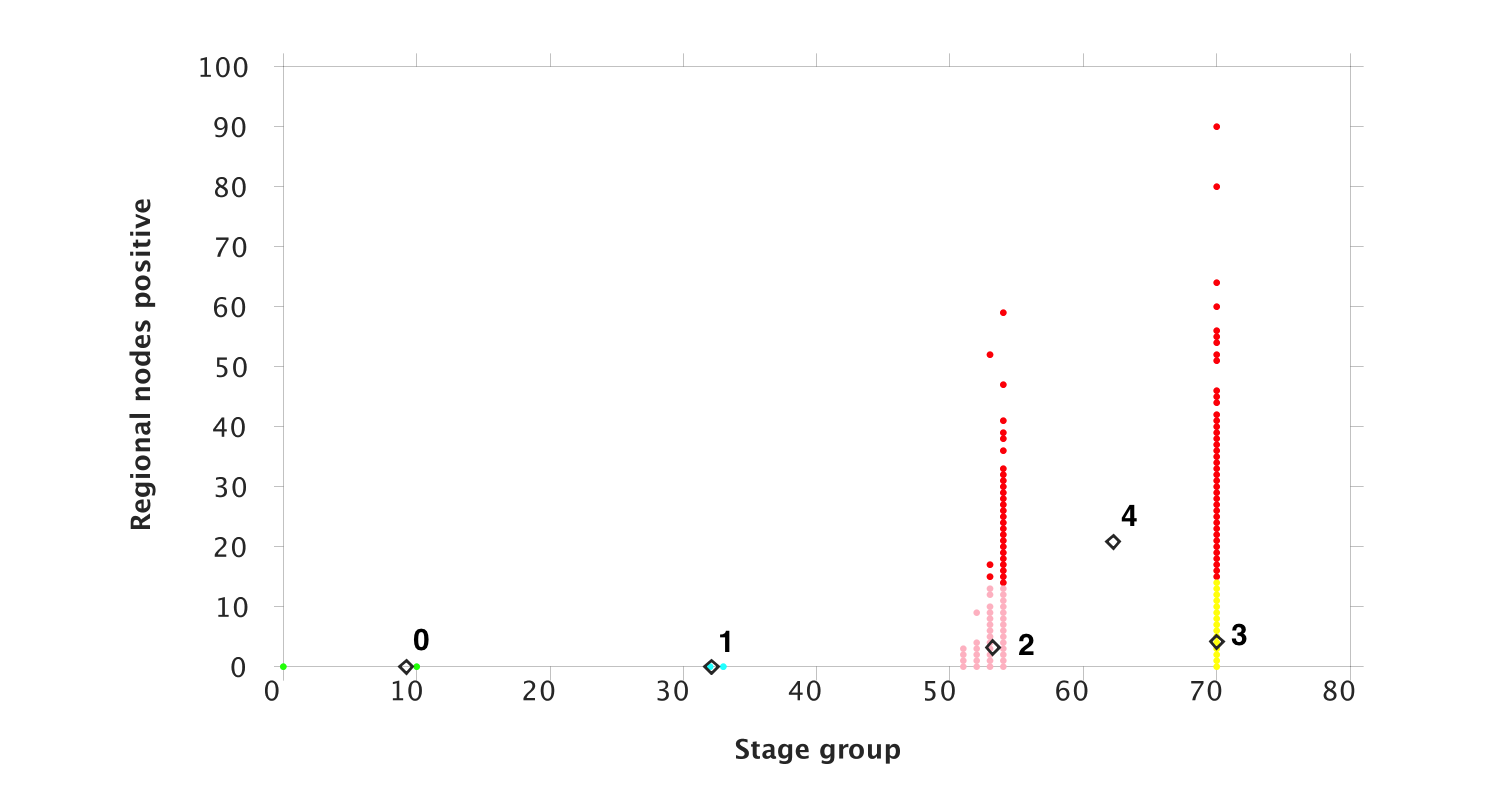### Bisecting K-means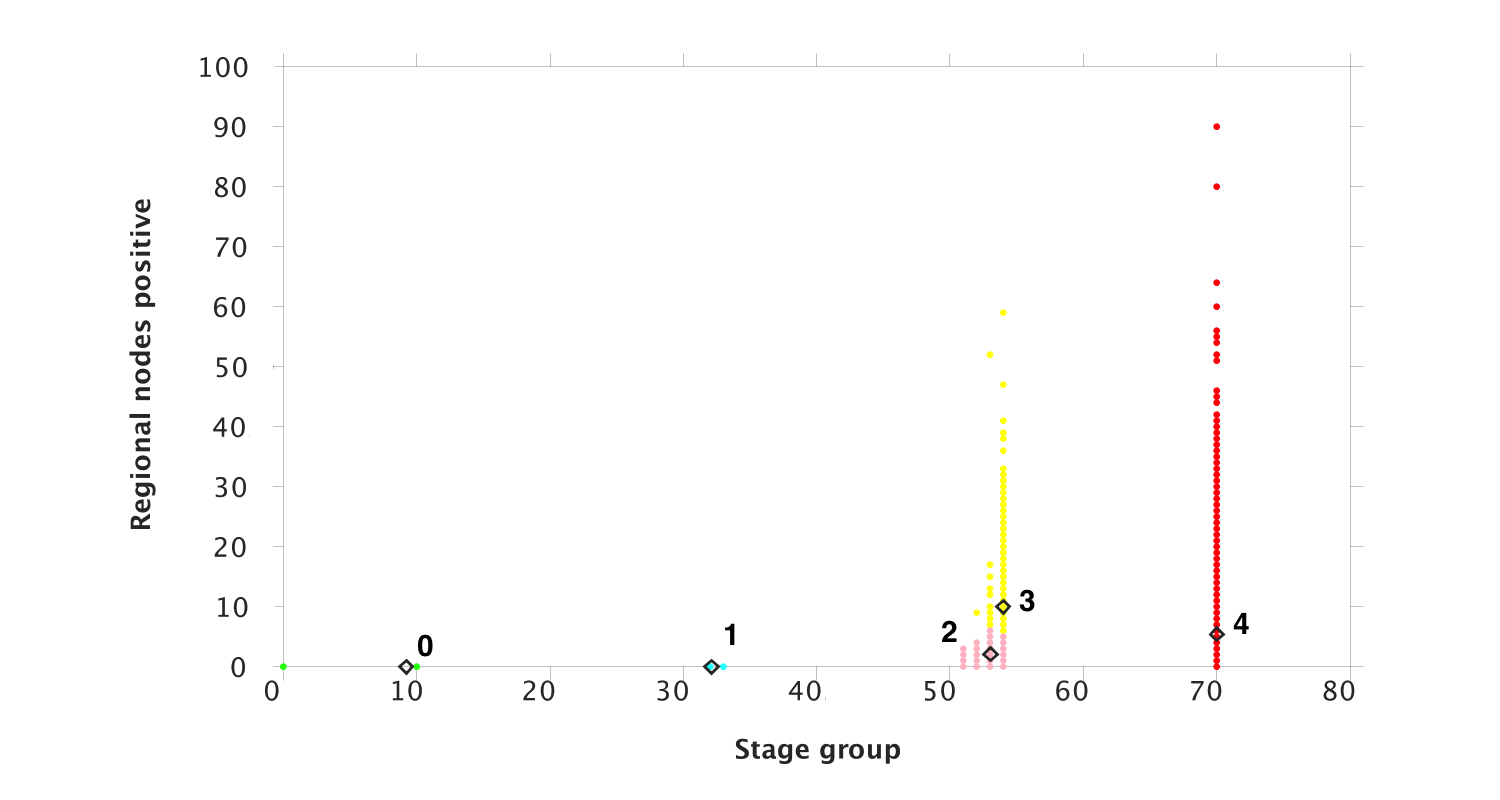### Gaussian Mixture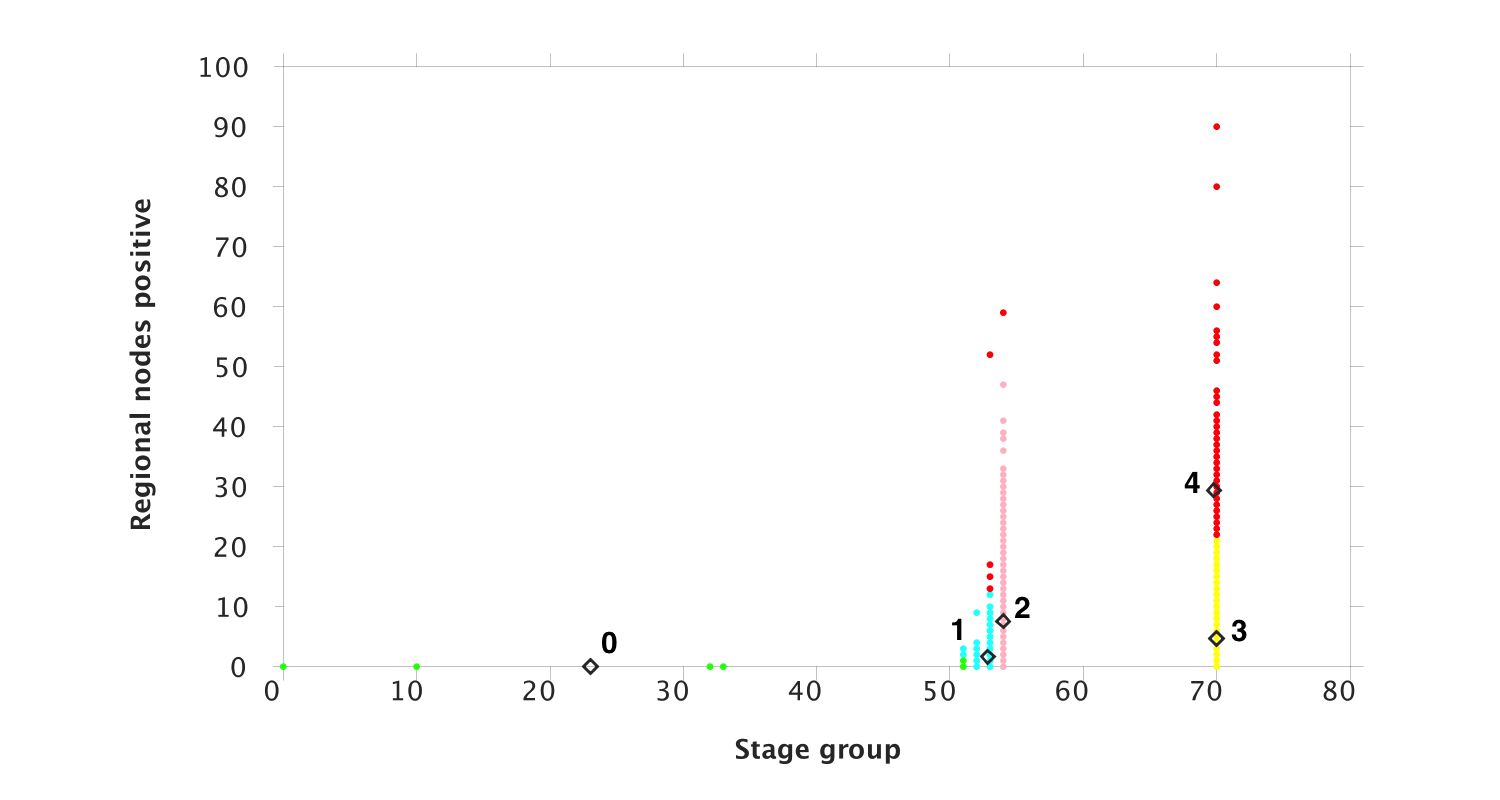### Observations

Each algorithm yielded a different set of clusters, however, common to all algorithms is the fact that average survival months for patients in a cluster decreases as the cluster center has increasing ‘regional nodes positive’ and ‘stage group’. (Recall that as the number indicating a cluster center increases, the average survival months for the patients in the cluster decreases.)

This is easy to see for each algorithm particularly for ‘stage group’. Regarding ‘regional nodes positive’, compare clusters 3 & 4 from K-means algorithm to observe that values of ‘regional nodes positive’ in cluster 4 are, in general, greater than those in cluster 3 and hence the average survival months drops. Same observation can be made by comparing clusters 2 & 3 from bisecting K-means algorithm and comparing clusters 3 & 4 from Gaussian mixture. This result is expected as increased stage and increased number of lymph nodes containing metastasis are both indicators of poor cancer prognosis.

## Concluding Remarks

In this article, we focused on clustering analysis with Apache Spark machine learning library MLlib and studied an example where K-means, bisecting K-means and Gaussian mixture algorithms are applied to colorectal cancer research data to explore patient data survival. As we will show in the next section, MLlib provides a simple API to apply those algorithms. Some additional comments are as follows.

• For any of the K-means, bisecting K-means and Gaussian mixture algorithms, it is not guaranteed that the algorithm would produce the same clusters if run multiple times. For example, we observed that running the K-means algorithm multiple times with the same parameters generated slightly different results at each run. In almost all cases WCSS was nearly identical and only once in a while, a particular run produced a WCSS which was significantly greater than others.
• Because the test setup consisted of a single-node Hadoop installation, i.e. not a multi-node cluster, we did not attempt to compare computational times between the algorithms. For a performance comparison between K-means and Gaussian mixture see Jung. et. al and cluster analysis lecture notes.
• In addition to K-means, bisecting K-means and Gaussian mixture, MLlib provides implementations of three other clustering algorithms, Power iteration clustering, Latent Dirichlet allocation and Streaming K-means. Those algorithms have APIs similar to the ones explored in this article.

## Code Review

The following is a class diagram of the code used in this article. Because the Spark MLlib API for K-means, bisecting K-means and Gaussian mixture algorithms are quite similar, we created a parent class named ClusteringColonCancerData to encapsulate all the common functionality. The subclasses KMeansClustering, BisectingKMeansClustering and GaussianMixtureClustering call the Spark MLlib K-means, bisecting K-means and Gaussian mixture APIs, respectively.##### Figure 5. Class diagram of the sample code.

The following table summarizes the main Spark MLlib API classes used in sample code.

 Package Class Description org.apache.spark SparkConf Provides configuration for a Spark application. org.apache.spark.api.java JavaSparkContext Java language implementation for a Spark context, main entry point for Spark functionality such as connecting to a Spark cluster and performing operations in it. org.apache.spark.api.java JavaRDD Java language implementation for the so-called resilient distributed dataset (RDD), a basic data structure in Spark for performing parallel operations in a cluster environment. org.apache.spark.mllib.clustering KMeans Implementation API for the K-means data clustering algorithm. org.apache.spark.mllib.clustering KMeansModel A data model representing one or more data clusters obtained via KMeans API. org.apache.spark.mllib.clustering BisectingKMeans Implementation API for the bisecting K-means data clustering algorithm. org.apache.spark.mllib.clustering BisectingKMeansModel A data model representing one or more data clusters obtained via BisectingKMeans API. org.apache.spark.mllib.clustering GaussianMixture Implementation API for the Gaussian mixture data clustering algorithm. org.apache.spark.mllib.clustering GaussianMixtureModel A data model representing one or more data clusters obtained via GaussianMixture API. org.apache.spark.mllib.stat.distribution MultivariateGaussian Represents an individual cluster in a GaussianMixtureModel object.

Table 3. Spark MLlib API classes used in sample code.

We used JCCKit in order to plot the graphs in this article. PropertyFileGenerator is a class that creates a property file from cluster data points that can be consumed by JCCKit libraries. Because it is unrelated to Apache Spark we do not review PropertyFileGenerator.

We executed our code in a Spark server with a single-node Hadoop installation, version 2.7.1 with Spark API version 1.6.1.

All the code is available from github.

### ClusteringColonCancerData

This is an abstract class responsible for the following main tasks.

• Initializing Spark configuration.
• Reading and parsing patient data file.
• Printing out cluster centers and related data, such as average survival months in each cluster.
• Stopping the Spark configuration after all the functionality is completed.

This class relies on its concrete subclasses to obtain the clusters.

The core functionality is performed by obtainClusters() that starts with initializing Spark configuration and context.

``````public abstract class ClusteringColonCancerData {

protected void obtainClusters(){
// Set application name
String appName = "ClusteringExample";

// Initialize Spark configuration & context
SparkConf sparkConf = new SparkConf().setAppName(appName)
.setMaster("local").set("spark.executor.memory", "1g");

JavaSparkContext sc = new JavaSparkContext(sparkConf);``````

Then, the patient data file is fetched from file system and parsed into a JavaRDD<String> data structure. Next, it is converted to a JavaRDD<Vector> data structure to be used by the algorithms. (We will look into mapFunction() used in conversion in a moment.)

``````        // Read data file from Hadoop file system.
String path = "hdfs://localhost:9000/user/konur/COLRECT.txt";

// Read the data file and return it as RDD of strings
JavaRDD<String> tempData = sc.textFile(path);

JavaRDD<Vector> data = tempData.map(mapFunction);

data.cache();``````

Define number of clusters and iterations. Then, call abstract method dataCenters() to perform the actual clustering task. The response from the method is two data structures, JavaRDD<Integer> clusterIndexes and Vector[] clusterCenters. The size of clusterCenters is equal to the number of clusters and each entry in that array gives the coordinates of the particular cluster center in terms of 'stage group' and 'regional nodes positive'. The size of clusterIndexes is the same as number of patients in patient data file and each entry in that data structure corresponds to a particular patient. Value of each entry is one of 0, …, #clusters - 1 to indicate which cluster the particular patient data belongs to. (Note that clusters are identified by an integer 0, …, #clusters - 1.) For example, if value of an entry in clusterIndexes is 3, then the corresponding patient record belongs to cluster 3.

``````        int numClusters = 5;

int numIterations = 30;

// Rely on concrete subclasses for this method.

Tuple2<JavaRDD<Integer>,Vector[]> pair = dataCenters(data,
numClusters, numIterations);

JavaRDD<Integer> clusterIndexes = pair._1();

Vector[] clusterCenters = pair._2();``````

Because Spark uses parallel processing across nodes, we bring the results to the driver node before processing them to display.

``````        // Bring all data to driver node for displaying results.
List<String> collectedTempData = tempData.collect();
List<Integer> collectedClusterIndexes = clusterIndexes.collect();``````

Finally, display the results. Then, stop and close the Spark context. (Review of displayResults() will follow in a moment.)

``````        // Display the results
displayResults(collectedTempData, collectedClusterIndexes,
clusterCenters);
sc.stop();
sc.close();
}``````

The conversion of patient data file from JavaRDD<String> to JavaRDD<Vector> is performed via mapFunction() below. The patient data file has data for one specific patient in each row, consisting of three integers separated by whitespace, ‘survival months’, ‘stage group’ and ‘regional nodes positive’. The call() method simply parses each line, places ‘stage group’ and ‘regional nodes positive’ into a double[] and adds to the return Vector.

``````    @SuppressWarnings("serial")
static Function<String, Vector> mapFunction =
new Function<String, Vector>() {
public Vector call(String s) {
String[] sarray = s.split(" ");
double[] values = new double[sarray.length - 1];
// Ignore 1st token, it is survival months and not needed here.
for (int i = 0; i < sarray.length - 1; i++)
values[i] = Double.parseDouble(sarray[i + 1]);
return Vectors.dense(values);
}
};``````

The utility method displayResults() prints out number of patients, average survival months in each cluster, data points (‘stage group’ and ‘regional nodes positive’) in each cluster together with how many times a data point is repeated, i.e. how many patients correspond to that particular ‘stage group’ and ‘regional nodes positive’. The size of collectedClusterIndexes is the same as number of patients. Each entry in collectedClusterIndexes corresponds to a particular patient.

``````    protected void displayResults(List<String> collectedTempData,
List<Integer> collectedClusterIndexes, Vector[] clusterCenters) {

System.out.println("\nTotal # patients: " +
collectedClusterIndexes.size());``````

An example output is as follows.

Total # patients: 30788

For each cluster center, the below data structure will contain the corresponding survival months in an ArrayList<Integer>. For each data point in the cluster, the corresponding survival months will be a distinct element in the ArrayList<Integer>.

``````Hashtable<Integer, ArrayList<Integer>> cl =
new Hashtable<Integer, ArrayList<Integer>>();``````

Start populating the data structures.

``````
int j = 0;

for (Integer i : collectedClusterIndexes) {
// This ArrayList<Integer> stores individual survival months
// for each data point.
ArrayList<Integer> srvMnths = cl.get(i);
if (srvMnths == null) {
srvMnths = new ArrayList<Integer>();
cl.put(i, srvMnths);
}

// For a data point, get the corresponding survival months,
// 'stage group' and 'regional nodes positive'.
String tempRow = collectedTempData.get(j++);
StringTokenizer strTok = new StringTokenizer(tempRow);
String survivalMonths = strTok.nextToken();
String stage = strTok.nextToken();
String regNodes = strTok.nextToken();

Define a data structure to store the number of times a unique pair of 'stage group' and 'regional nodes positive' is encountered. The key is a vector with two elements: 'stage group' and 'regional nodes positive'.

``````            Hashtable<java.util.Vector<Integer>, Integer> dataPoints =
clusteredPoints
.get(i);

if (dataPoints == null) {
dataPoints = new Hashtable<java.util.Vector<Integer>,
Integer>();
clusteredPoints.put(i, dataPoints);
}

// Construct a vector consisting of a unique pair of
// 'stage group' and 'regional nodes positive'.
java.util.Vector<Integer> pnt = new java.util.Vector<Integer>();

// Have we encountered with that unique pair of 'stage group'
// and 'regional nodes positive' before?
Integer numOccurences = dataPoints.get(pnt);

if (numOccurences == null) {
dataPoints.put(pnt, 1);
}
// If answer is yes, increment the # times we encountered with
// that particular pair of 'stage group' and 'regional nodes
// positive'.
else {
dataPoints.put(pnt, numOccurences + 1);
}

}``````

Now, we can display the average survival months and # data points in each cluster. (We will look into method avg() in a moment.)

``````        Enumeration<Integer> keys = cl.keys();
while (keys.hasMoreElements()) {
Integer i = keys.nextElement();
System.out.println("\nCluster " + i);
System.out.println("# points: " + cl.get(i).size());
System.out.println("Average survival months: " + avg(cl.get(i)));
}``````

An example output is as follows.

Cluster 1

# points: 9660

Average survival months: 65.91252587991718

For each cluster display distinct pair of 'stage group' and 'regional nodes positive' and how many times they occurred.

``````        Enumeration<Integer> keysPoints = clusteredPoints.keys();
while (keysPoints.hasMoreElements()) {
Integer i = keysPoints.nextElement();
System.out.println("\nCluster " + i + " points:");
Hashtable<java.util.Vector<Integer>, Integer> dataPoints =
clusteredPoints
.get(i);
Enumeration<java.util.Vector<Integer>> keyVectors = dataPoints
.keys();
while (keyVectors.hasMoreElements()) {
java.util.Vector<Integer> pnt = keyVectors.nextElement();
System.out.println("[ 'stage group': " + pnt.get(0)
+ ", 'regional nodes positive': " + pnt.get(1) + "]"
+ "  repeated " + dataPoints.get(pnt) + " time(s). ]");
}
}``````

An example output is as follows.

Cluster 1 points:

[ 'stage group': 32, 'regional nodes positive': 0]  repeated 8477 time(s). ]

[ 'stage group': 33, 'regional nodes positive': 0]  repeated 1183 time(s). ]

Using the helper class PropertyFileGenerator, generate a property file to be used by JCCKit for plotting the clusters and corresponding data points.

``````        PropertyFileGenerator.generatePropertyFileForGraph(clusteredPoints,
clusterCenters);
}``````

This completes the displayResults() method.

The method avg() iterates through its input parameter to obtain a sum and then calculate the average.

``````   private static double avg(ArrayList<Integer> in) {
if (in == null || in.size() == 0) {
return -1.;
}

double sum = 0.;
for (Integer i : in) {
sum += i;
}
return (sum / in.size());
}``````

The signature for abstract method dataCenters() is as follows.

``````    protected abstract Tuple2<JavaRDD<Integer>,Vector[]>
dataCenters(JavaRDD<Vector> data, int numClusters,
int numIterations);``````

We will now review how each individual subclass implements that method, to calculate clusters according to the particular algorithm.

## KMeansClustering

The main method creates an instance and calls the parent method obtainClusters().

``````public class KMeansClustering extends ClusteringColonCancerData {

public static void main(String[] args) {
KMeansClustering kMeans = new KMeansClustering();
kMeans.obtainClusters();
}``````

Implementation of abstract method dataCenters() starts with calling train() on org.apache.spark.mllib.clustering.KMeans passing to it data, number of clusters and number of iterations. This will yield an org.apache.spark.mllib.clustering.KMeansModel object.

``````    public Tuple2<JavaRDD<Integer>,Vector[]> dataCenters(JavaRDD<Vector> data,
int numClusters,
int numIterations){
// Obtain model
KMeansModel clusters = KMeans.train(data.rdd(), numClusters,
numIterations);``````

Evaluate and display WCSS.

``````        double WCSS = clusters.computeCost(data.rdd());
System.out.println("WCSS = " + WCSS);``````

An example output is as follows.

WCSS = 279001.8087726742

Now display cluster centers.

``````        Vector[] clusterCenters = clusters.clusterCenters();
for (int i = 0; i < clusterCenters.length; i++) {
Vector clusterCenter = clusterCenters[i];
double[] centerPoint = clusterCenter.toArray();
System.out.println("Cluster Center " + i + ": [ 'stage group': "
+ centerPoint +
", 'regional nodes positive': " + centerPoint + " ]");
}``````

An example output is as follows.

Cluster Center 1: [ 'stage group': 32.12246376811594, 'regional nodes positive': 0.0 ]

Finally, call predict() to obtain the data structure to be returned from the method.

``````        JavaRDD<Integer> clusterIndexes = clusters.predict(data);

Tuple2<JavaRDD<Integer>,Vector[]> results =
new Tuple2<JavaRDD<Integer>,Vector[]>(clusterIndexes, clusterCenters);
return results;
}
}``````

This completes the review of KMeansClustering.

## BisectingKMeansClustering

This is very similar to KMeansClustering. The main method creates an instance and calls the parent method obtainClusters().

``````public class BisectingKMeansClustering extends ClusteringColonCancerData {
public static void main(String[] args) {
BisectingKMeansClustering bisectingKMeans =
new BisectingKMeansClustering();
bisectingKMeans.obtainClusters();
}
``````

Implementation of abstract method dataCenters() starts with creating an instance of org.apache.spark.mllib.clustering.BisectingKMeans and configuring it with number of clusters and number of iterations.

``````    public Tuple2<JavaRDD<Integer>,Vector[]> dataCenters(JavaRDD<Vector> data,
int numClusters,
int numIterations){
BisectingKMeans bkm = new BisectingKMeans().setK(numClusters)
.setMaxIterations(numIterations);``````

Then, call the run() method to obtain org.apache.spark.mllib.clustering.BisectingKMeansModel.

``        BisectingKMeansModel clusters = bkm.run(data);``

Evaluate and display WCSS.

``````        double WCSS = clusters.computeCost(data.rdd());
System.out.println("WCSS = " + WCSS);
``````

Display cluster centers.

``````        Vector[] clusterCenters = clusters.clusterCenters();
for (int i = 0; i < clusterCenters.length; i++) {
Vector clusterCenter = clusterCenters[i];
double[] centerPoint = clusterCenter.toArray();
System.out.println("Cluster Center " + i
+ ": [ 'stage group': " + centerPoint +
", 'regional nodes positive': " + centerPoint + " ]");
}``````

Finally, call predict() to obtain the data structure to be returned from the method.

``````        JavaRDD<Integer> clusterIndexes = clusters.predict(data);

Tuple2<JavaRDD<Integer>,Vector[]> results =
new Tuple2<JavaRDD<Integer>,Vector[]>(
clusterIndexes, clusterCenters);
return results;
}
}``````

This completes the review of BisectingKMeansClustering.

## GaussianMixtureClustering

The main method creates an instance and calls the parent method obtainClusters().

``````public class GaussianMixtureClustering extends ClusteringColonCancerData {

public static void main(String[] args) {
GaussianMixtureClustering gaussianMixture =
new GaussianMixtureClustering();
gaussianMixture.obtainClusters();
}``````

Implementation of abstract method dataCenters() starts with creating an instance of org.apache.spark.mllib.clustering.GaussianMixture and configuring it with number of clusters and number of iterations.

``````
public Tuple2<JavaRDD<Integer>, Vector[]> dataCenters(JavaRDD<Vector> data,
int numClusters, int numIterations) {
GaussianMixture gm = new GaussianMixture().setK(numClusters)
.setMaxIterations(numIterations);``````

Then, call the run() method to obtain org.apache.spark.mllib.clustering.GaussianMixtureModel.

``        GaussianMixtureModel clusters = gm.run(data.rdd());``

Having obtained the model, display cluster centers similarly to the other two algorithms.

``````        Vector[] clusterCenters = new Vector[clusters.gaussians().length];
int i = 0;

MultivariateGaussian[] gaussians = clusters.gaussians();
for (MultivariateGaussian mg : gaussians) {
Vector clusterCenter = mg.mu();
clusterCenters[i] = clusterCenter;
double[] centerPoint = clusterCenter.toArray();
System.out.println("Cluster Center " + i
+ ": [ 'stage group': " + centerPoint +
", 'regional nodes positive': " + centerPoint + " ]");
i++;
}``````

Finally, call predict() to obtain the data structure to be returned from the method.

``````        JavaRDD<Integer> clusterIndexes = clusters.predict(data);

Tuple2<JavaRDD<Integer>, Vector[]> results =
new Tuple2<JavaRDD<Integer>, Vector[]>(
clusterIndexes, clusterCenters);
return results;

}
}
``````

This completes the review of GaussianMixtureClustering. (Please note that Gaussian mixture API does not readily provide a method to calculate WCSS.)

Topics:
apache spark, big data analytics, clustering, machine learning, unsupervised learning, medical research, cancer

Opinions expressed by DZone contributors are their own.